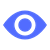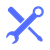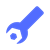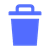# Services API - Calculated metrics### List all calculated metrics

Get an overview of all calculated metrics.### View a calculated metric

Get the descriptor of a calculated metric by its ID.### Create a calculated metric

Create a new calculated metric with the exact parameters you need.### Edit a calculated metric

Update an existing calculated metric. You can also create a new calculated metric with the specified ID.### Delete a calculated metric

Delete calculated metrics you don't need anymore.